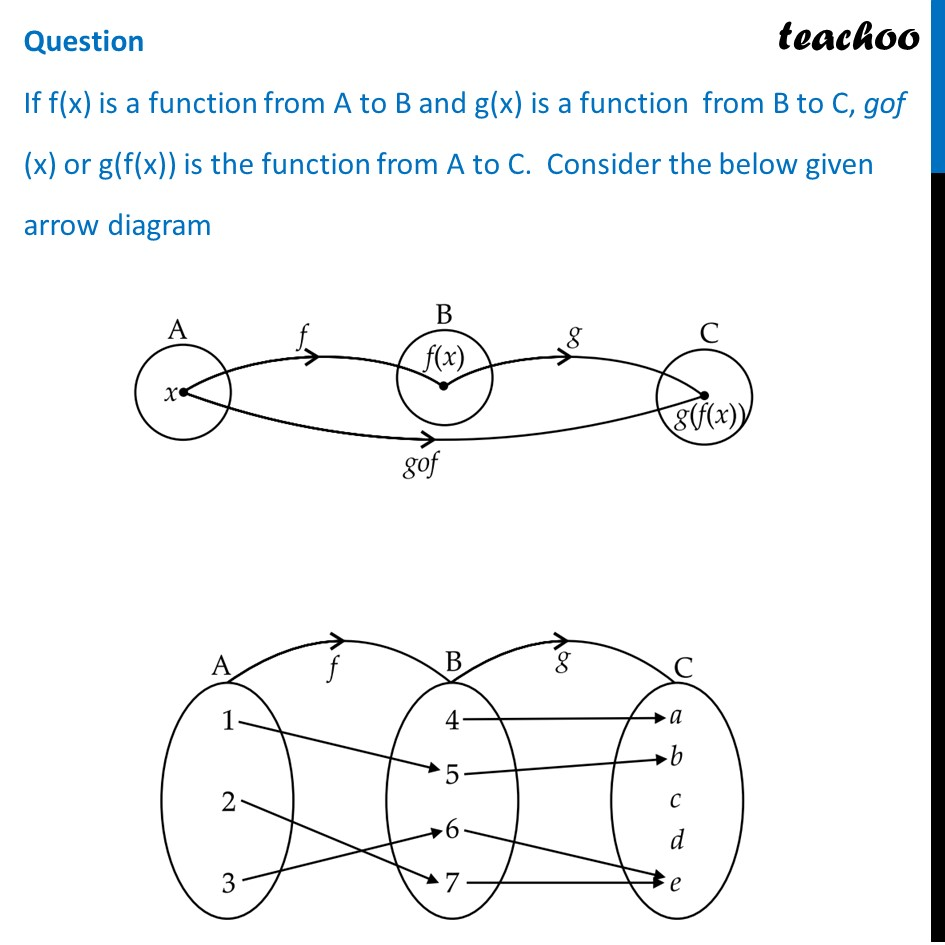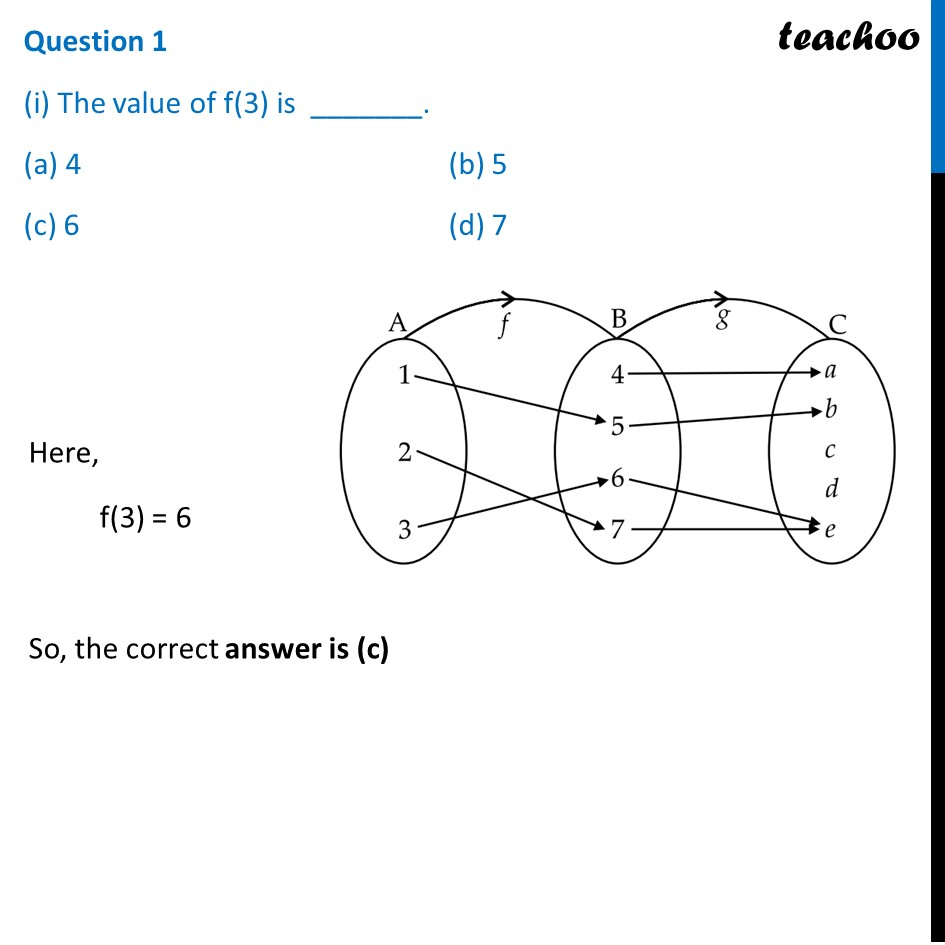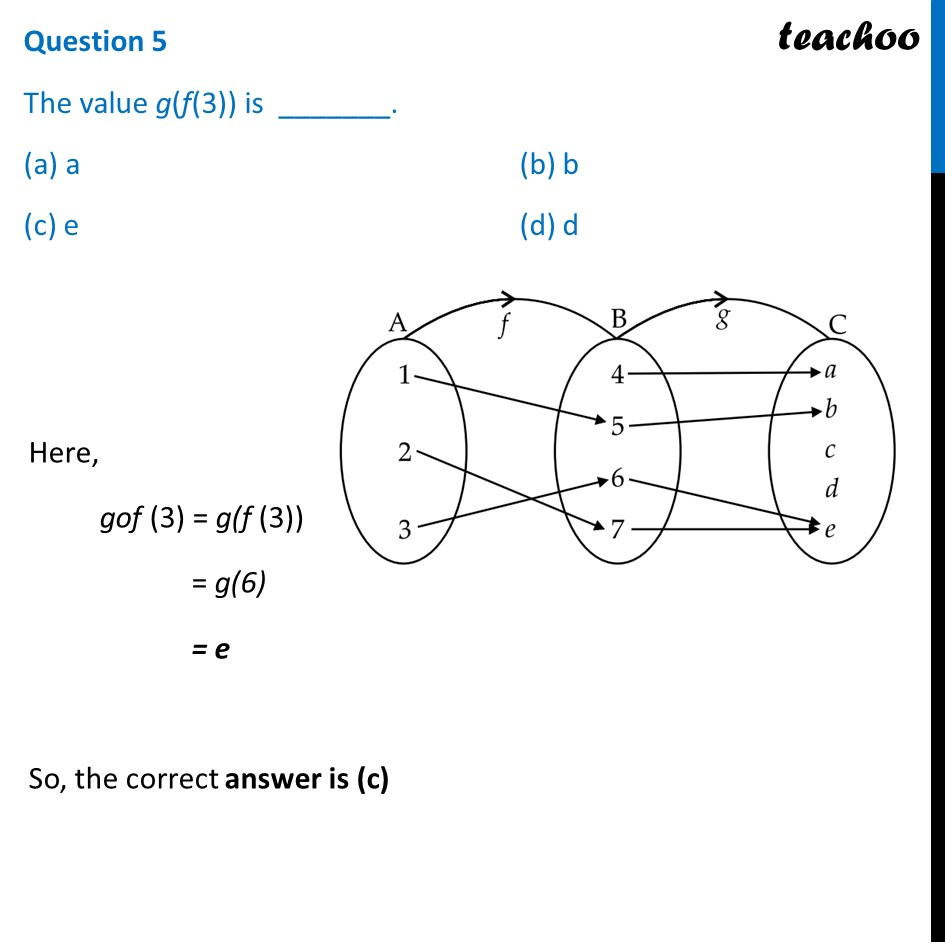## Question If f(x) is a function from A to B and g(x) is a function  from B to C, gof (x) or g(f(x)) is the function from A to C. Consider the below given arrow diagram## (i) The value of f(3) is  _______.  (a) 4   (b) 5  (c) 6   (d) 7##1. Chapter 1 Class 12 Relation and Functions (Term 1)
2. Serial order wise
3. Case Based Questions (MCQ)

Transcript

Question If f(x) is a function from A to B and g(x) is a function from B to C, gof (x) or g(f(x)) is the function from A to C. Consider the below given arrow diagram Question 1 (i) The value of f(3) is _______. (a) 4 (b) 5 (c) 6 (d) 7 Here, f(3) = 6 So, the correct answer is (c) Question 2 The value of g(5) is _______. (a) a (b) b (c) c (d) d Here, g(5) = b So, the correct answer is (b) Question 3 The value of gof (2) is _______. (a) a (b) b (c) d (d) e Here, gof (2) = g(f (2)) = g(7) = e So, the correct answer is (d) Question 4 The function f(x) is _______. (a) one-one and onto (b) one-one, but not onto (c) onto, but not one-one (d) neither one-one nor onto One-one Since each of 1, 2, 3 have a different image in B ∴ f(x) is one-one Onto Since all elements B do not have a pre-image in A ∴ R is not onto So, the correct answer is (B) Question 5 The value g(f(3)) is _______. (a) a (b) b (c) e (d) d Here, gof (3) = g(f (3)) = g(6) = e So, the correct answer is (c)